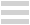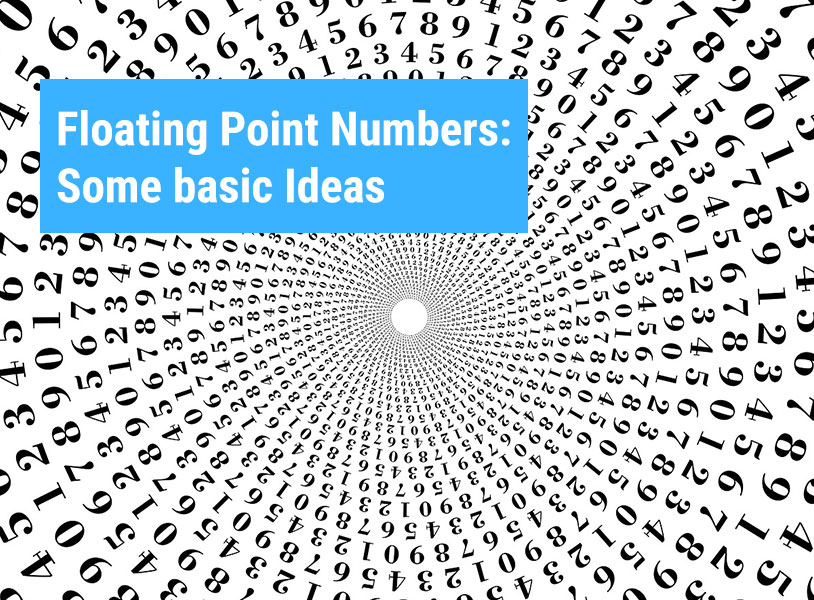# Floating Point Numbers: Some basic IdeasStudents generally come across this term “float” in their computer programming (mainly C, C++ and Java) courses. For variable declaration prior to a code, certain data types are used like integer types (int, char, short etc.), floating point types (float) etc. Students generally have the notion that for decimal values, the “float” data type is to be used. This is correct but there are other data types present as well under the floating point category having minor differences from the “float” data type. These other ones include “double” and “long double” in C. Let's go through some basic ideas related to the floating point numbers that are useful in both mathematics as well as programming.

## Floating Point Numbers - Introduction

Definition

A number which contains one fractional (post-decimal) part where there's no limitation on the number of digits after the decimal point is called a floating point number. Some examples are 3.0, -25.52, 15.567845321 etc.

Why is it referred to as “floating point”?

It's a known fact that there's no fixed number of digits after as well as before a decimal point. Therefore, that decimal point is said to “float” among the digits. Thus, it's referred to as a “floating point”.

Let's take the number 12.34 as an example. It's a floating point number. The number of digits after and before a decimal is not fixed. The number 0012.340 is the same as 12.34 where the number of digits after as well as before that decimal point has increased.

A point to be noted here is that most of the floating point numbers represented by computers are merely approximations. The main challenge in programming using floating point numbers is to make sure that the approximated values provide a reasonably correct result. Mathematics involving floating point numbers require good computational power. Therefore, certain microprocessors have a chip called FPU (Floating Point Unit) that specializes in operations using floating point numbers.

## Why is there a need for Floating Point Numbers?

Due to the limitations of computer memory, it's pretty difficult to store numbers in the computer with limitless precision. It doesn't matter whether binary fractions are used or decimals are used. There's a limit on the number of digits at some definite point. Therefore the result of certain calculations cannot be deemed accurate. Consider these three cases:

• An engineer is involved in the project of construction of a highway. It's of little importance here whether the width of the highway is 20m or 20.01m. A relative accuracy here would work fine.

• A designer is involved in designing microchips for computers. For designing microchips, a simple one-tenth of a millimeter (0.0001m) is a massive difference. But the measurements are usually based only on lower magnitudes as the designer generally does not have to be concerned with any distance greater than 0.1m.

• A physicist who's involved in a calculation that requires the values of Newton's gravitational constant (6.674×10^−11 N⋅m2/kg approx.) and the speed of light (3.00×10^8 m/s approx.).

In case of the engineer and the designer, a certain number format is required that can provide accuracy at different magnitudes (either higher or lower). But in case of the physicist, the calculation involved contains numbers at both the higher as well as the lower magnitude.

Hence, it can be concluded that a fixed number of fractional digits and integers is not always useful. The only solution should be a format where floating point numbers are used where the number of digits after and before a decimal is not fixed.

## How Floating Point Numbers work

Floating Point Numbers compose a specific number into two parts:

a) Significand- It contains the significant digits of the number. Negative numbers are represented by negative significands.

b) Exponent- The exponent in case of floating point numbers is the power of the base (10). Numbers that are very close to 0 are represented by negative exponents. The exponent can be written with the inclusion of the base (10) or it can be written with an 'E' that separates it from significand.

Note: The decimal point in floating point numbers is placed between the first and the second digit of the significand.The above format can:

• Represent various numbers at extremely different magnitudes.

• Provide same accuracy (relatively) at almost all magnitudes.

• Allow relatively accurate calculations across numbers of different magnitudes (like multiplication of a huge number with an extremely small number).

## Floating Point Types in C

The table below will provide you with the details like storage sizes, value ranges as well the precisions of floating-point types:The main advantage of floating point numbers over fixed point numbers is that the limitation of digits after the decimal points is lifted. Hence, it can handle a larger range of numbers compared to that of the fixed point types. But there's one disadvantage as well. Floating point numbers consume more system storage spaces than fixed point numbers to accommodate the large number of digits after the decimal point.### FUTURITE

Futurite aims to bring high quality STEM (Science, Technology, Engineering and Mathematics) education to school students in India. Our mission is to use cutting-edge technologies such as Robotics and 3D Printing to impart a thorough grounding in STEM subjects and concepts to school students while making their learning experience fun, engaging and hands-on. Futurite has been operating in Dubai successfully (as Premier Genie) since a number of years, working with over 25 of the top international schools and teaching 1000+ students. Futurite has been founded by alumni from IIT, IIM, JU and HEC Paris.